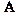# Triangulator triangle calculatorVersion Française

 Side A = B = C = Opposite angle in   d =   e =   f = Perimeter =   Surface =

Results

Symbolic

A, B, C = length of sides

d, e, f = angles, opposite to sides A, B, C

P = Perimeter , S = Surface

# triangle solution, résolution, triangulateur, calculateur de triangle, solution de triangle, calcul triangle, calc

### How to     ( Javascript must be enabled )

Input 3 values including at least one non angular one.

( d is the angle opposite to side A; e opposite to B; f opposite to C

Click button [ Calculate ] or [ Enter ] key to get all other values and a drawing of that triangle.

Or click in the white left zone to directly draw a triangle.

The 3 inputs used for solution will be shaded in yellow background.

[ Rotation ] to turn clockwise the triangle on another side.

[ Miror ] to reverse the triangle left to right.

[ Reset] or [ Esc ] key to erase all values.

[ Data ] leaves only input data. ( Note the two blanks preceding calculated values. )

[ Complementary ] gives a second possible solution.

[ degree ] or [ radiant ] to change angular unit.

[ < ] or [ > ] ( at top with Triangulator # ) to go backward or forward to preceding solutions.

Scientific notation may be used. For exemble .0000123 will be presented 1.23e-5 = 1.23×10-5.

The calculation is done with the three first inputs entered according to the order A B C d e f P S.

Others values are discarded and not concorded.

An impossible calculation warning can be announced with an error # specified in the Symbolic box.

Immediately after a successful calculation, you can change the number of decimal places by clicking on the [ + ] or [ - ] button.

< The text in Results box can be copied and pasted to be saved elsewhere.

Pasting others results there, [ Report results ] inputs them in the calculator.

Ref: Wikipedia + Solution

by Richard Lefebvre

rl@rlefebvre.ca

# triangle solution solve triangulator calculator perimeter surface area inscribed circumscribed